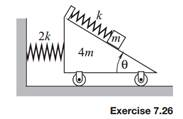### Create an Account

Home / Questions / The stiffness of the horizontal spring is 2k whereas the spring holding the small block ha...

# The stiffness of the horizontal spring is 2k whereas the spring holding the small block has stiffness k The masses are 4m and m for the cart and the block respectively Frictional resistance at all

The stiffness of the horizontal spring is 2k, whereas the spring holding the small block has stiffness k. The masses are 4m and m for the cart and the block, respectively. Frictional resistance at all contacting surfaces is negligible. Determine the equations of motion for the system.Jun 16 2020 View more View LessSubscribe To Get Solution